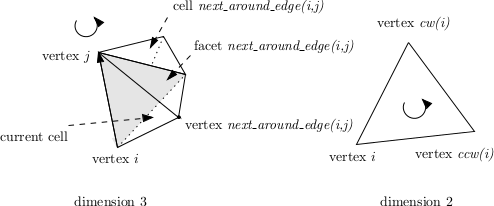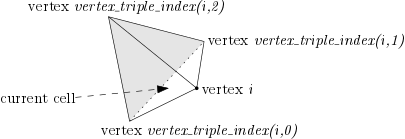CGAL 5.4 - 3D Triangulation Data Structure
CGAL::Triangulation_utils_3 Struct Reference

#include <CGAL/Triangulation_utils_3.h>

## Definition

The class Triangulation_utils_3 defines operations on the indices of vertices and neighbors within a cell.Figure 46.1 Operations on indices.

## Operations

static unsigned int next_around_edge (unsigned int i, unsigned int j)
In dimension 3, index of the neighbor n that is next to the current cell, when turning positively around an oriented edge whose endpoints are indexed i and j. More...

static int vertex_triple_index (const int i, const int j)
In dimension 3, index of the j'th vertex in counterclockwise order on the face opposite to vertex with i of the cell. More...

static unsigned int ccw (unsigned int i)
Has a meaning only in dimension 2. More...

static unsigned int cw (unsigned int i)
Same for clockwise.

## ◆ ccw()

 static unsigned int CGAL::Triangulation_utils_3::ccw ( unsigned int i )
static

Has a meaning only in dimension 2.

Computes the index of the vertex that is next to the vertex numbered i in counterclockwise direction. (see Figure 46.1).

Precondition
i<3.

## ◆ next_around_edge()

 static unsigned int CGAL::Triangulation_utils_3::next_around_edge ( unsigned int i, unsigned int j )
static

In dimension 3, index of the neighbor n that is next to the current cell, when turning positively around an oriented edge whose endpoints are indexed i and j.

According to the usual numbering of vertices and neighbors in a given cell, it is also the index of the vertex opposite to this neighbor n. (see Figure 46.1).

Precondition
( i < 4 ) && ( j < 4 ) && ( i != j ).

## ◆ vertex_triple_index()

 static int CGAL::Triangulation_utils_3::vertex_triple_index ( const int i, const int j )
static

In dimension 3, index of the j'th vertex in counterclockwise order on the face opposite to vertex with i of the cell.

Precondition
( i < 4 ) && ( j < 3 ).